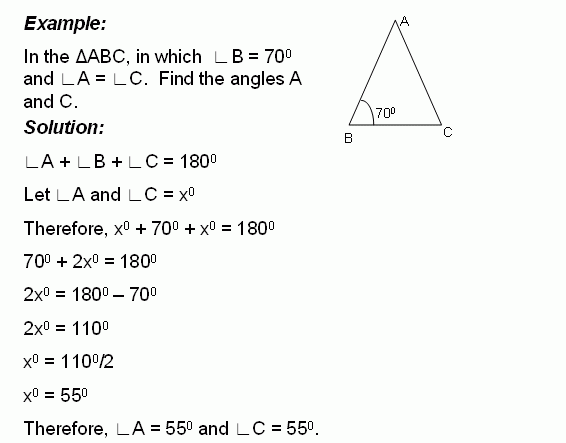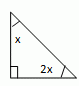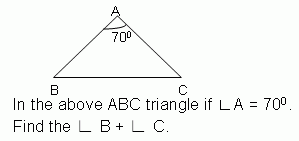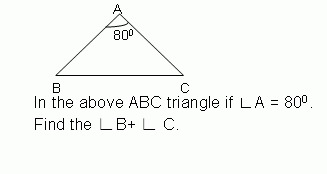Email us to get an instant 20% discount on highly effective K-12 Math & English kwizNET Programs!

#### Online Quiz (WorksheetABCD)

Questions Per Quiz = 2 4 6 8 10

### Middle/High School Algebra, Geometry, and Statistics (AGS)6.14 Find the Angles of TriangleDirections: Solve the following problems. Also write at least 10 examples of your own.
 Q 1:95 degrees90 degrees100 degrees Q 2: Find the angles of the triangle and classify based on the angles.30, 60, right angle triangle26, 64, right angle triangle32, 96, right angle triangle Q 3: Find the angles of the triangle.30, 60, 90 triangle45, 45, 90 triangle60, 60, 60 triangle Q 4: The measure of angle x is _____ degrees.Answer: Q 5:60 degrees70 degrees65 degrees Q 6:110 degrees100 degrees105 degrees Q 7:65 degrees75 degrees60 degrees Q 8:160 degrees75 degrees100 degrees Question 9: This question is available to subscribers only! Question 10: This question is available to subscribers only!